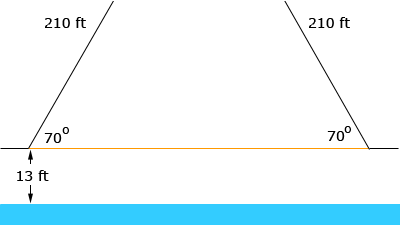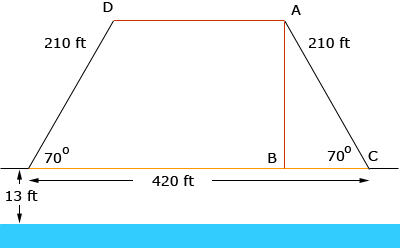i am a 10th grade student named trenae with a secondary question about the angles elevation. the waterway between lake huron and lake superior separates the u.s and canada.it is usually 13 feet above the water when its closed and each section is 210 feet long if the angle of elevation is 70 degrees then what is the distance from the top of the drawbridge to the water and the width of the gap created by the 2 sections of the bridge Trenae, Here is what I see from the situation you describe.The bridge is up and the orange line shows where the bridge surface is when it is closed. Now I want to add some lines and label some points on the diagram.Since triangle ABC is a right triangle you can use the sine and cosine of 70o to find the lengths of AB and BC. Can you use this to complete the problem? Penny